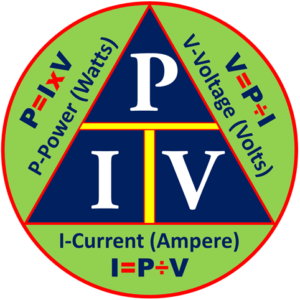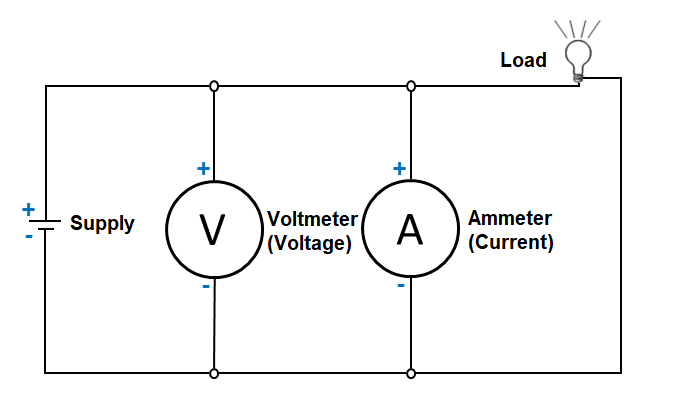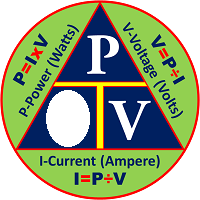## The Current Triangle Formula

The Power, Current, Voltage Formula TriangleHow do we use the formula triangle?

When we use the triangles to solve the unknown values, the given equivalent figure on the apex of the triangle is divided by either of the values at the base of the the triangle, whichever is the given meter reading or electronic value. To find the value of the apex, a given value at either side of the base of the triangle is multiplied by the other base value.

Let’s start now. In this lesson we will practice using this in calculating the electrical current that runs in a circuit.

The Current Triangle FormulaTo find the current that flows in a circuit, cover the “I” in the triangle and use the resulting equation. Using the circuit above, let us assume that the voltage is 4 volts, and the power consumption was known to be 20 watts. Calculate the circuit current.Summary: The electricity formula wheel is a combination or compilation of the different triangle formulas that provides solutions to solving or calculating the resistance, current, voltage and power consumption of an electrical circuit.

When we use the triangles to solve the unknown values, the given equivalent figure on the apex of the triangle is divided by either of the values at the base of the the triangle, whichever is the given meter reading or electronic value. A given value at either side of the base of the triangle is multiplied by the other base value, and vice versa.

Posted in